STATICALLY INDETERMINATE PROBLEMS IN TENSION AND COMPRSSION 2

# 1STATICALLY INDETERMINATE PROBLEMS IN TENSION AND COMPRSSION 2

## 1.1 Problem definition

Determine the axial forces in all three beams which are coupled at point D and submitted with a vertical downward load: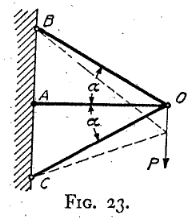Figure 2: Model by Timoshenko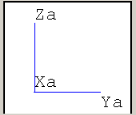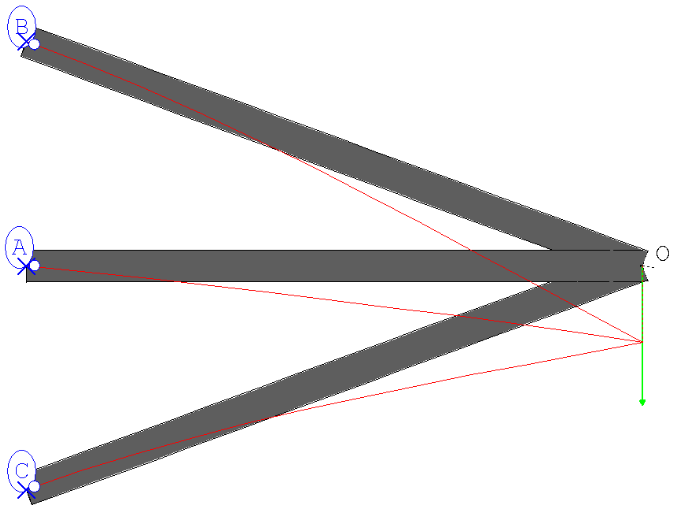Figure 1: Rohr2 Model

## 1.2 References (Timoshenko)

S. Timoshenko, Strength of Material, Part I, Elementary Theory and Problems, 3rd Edition, D. Van Nostrand Co., Inc., New York, NY, 1956, Chapter 1.6, pg. 25.

System consists of three bars, which are connected at the point O. The following parameters are given:

• Modulus of Elasticity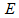• Force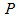• Length of each beam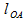,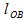,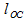• Angle of inclination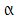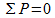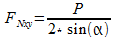Where:

 Variable Description Unit Used Value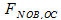Tensile Force; Compressive Force lbf 7309,51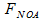Normal force lbf 0,00Vertical load lbf 5000,00Angle of inclination deg 20,00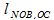Length OB,OC inch 63,85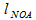Length OA inch 60,00

Table 1: Overview of the variables used

## 1.3 Model description (ROHR2)

In the model three beams are attached to the wall at the points A,B, and C one side and coupled together at the other end at point O. The system is symmetric about the middle, horizontal beam. They upper and lower beams are inclined with a given angle Î±. At the node O, a point load P of 5000 lbf was entered. All ends of the beams are hinged so that all beams are tension bars. In the model at every end of the beams a hinge is inserted, to ensure that the moment (Mx) isn't transmitted. For this system a profile with an outer diameter of 80 mm made of carbon steel is used. As the gravitational acceleration should not be taken into account, an additional load case (occasional loads; Load1) is defined which contains only the force at point O.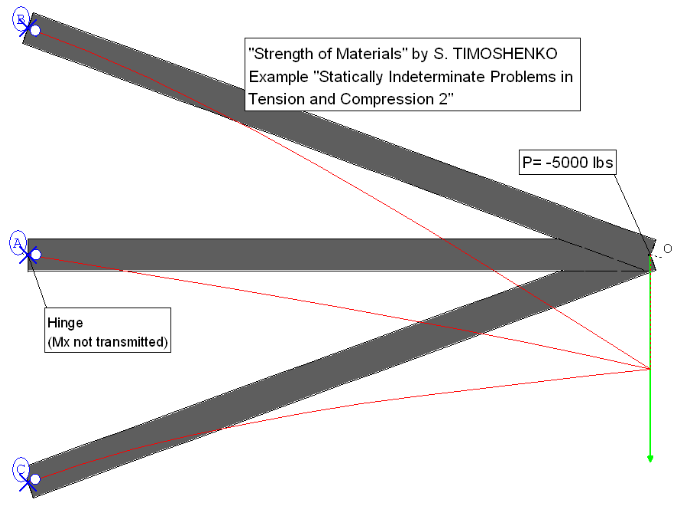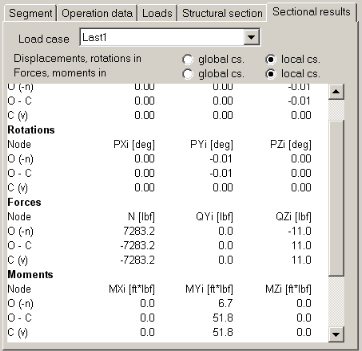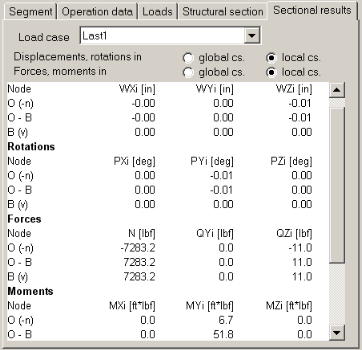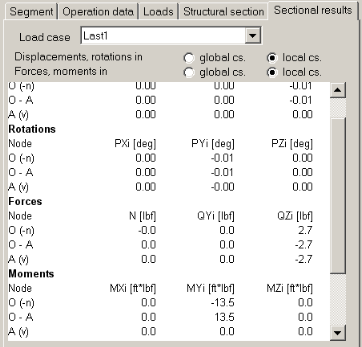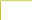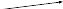Figure 3: FN at every beam

## 1.4 Result comparisons

 Value Length [inch] Reference (Timoshenko) [lbf] Rohr2 [lbf] Difference [%]63,85 7309,51 7283,2 <0,3760,00 0,00 0,0 <0,01

Table 2: Comparison of the axial forces for each beam

## 1.5 Conclusion

The results are satisfactorily, because the differences are very small. The difference are within the precision expected, given the number of digits in the input.

It can be remarked, that the dimensions, length, loads and materials are freely selectable, as the have no influence on the results checked in the example given by Timoshenko. He emphasizes the equality of loads at the beams BO and CO, where the beam BO experiences tension force and the beam CO experiences compressive axial force. The normal forces at beam OA is also equal to zero. Both requirements are exactly fulfilled.

## 1.6 Files

R006_inch.r2w

R006_mm.r2w

R2_stresses_6.ods

SIGMA Ingenieurgesellschaft mbH www.rohr2.com